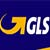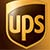# DIGISAT 122 Expert

[5 mm / 0,197 inches Cable]

75 Ohm DIGITAL SATELLITE CABLE in A Class specifically designed for applications such as digital television (DVB-T) and satellite reception.

Available in 5 different colours: white, black, blue, red and green.

##avviso75ohm##

SELECT AN AVAILABLE OPTION
 Choose packaging SELECT 40 meters 82 meters (blue) 90 meters Blue 100 meters 105 meters 110 meters (blue) 112 meters (blue) 120 meters Blue 125 meters 127 meters 130 meters 135 meters (blue) 140 meters Blue 145 meters 30 meters Green 35 meters (green) 39 meters 40 meters 42 meters 46 meters 50 meters (green) 90 meters (green) 105 meters Green 106 meters 108 meters 110 meters 115 meters Green 125 meters 130 meters Green 135 meters Green 140 meters 145 meters 37 meters 43 meters 45 meters 55 meters Red 62 meters 70 meters 72 meters 100 meters Red 115 meters 120 meters 122 meters Red 125 meters Red 140 meters 145 meters Red 85 meters 86 meters 90 meters 100 meters 52 meters 99 meters 71 meters 79 meters 46 meters 101 meters 104 meters 105 meters 110 meters 114 meters 116 meters 117 meters 118 meters 119 meters 121 meters 123 meters 124 meters 126 meters 127 meters 129 meters 130 meters 132 meters 133 meters 134 meters 135 meters 136 meters 137 meters 139 meters 142 meters 149 meters 155 meters 157 meters 158 meters 160 meters 161 meters 164 meters 165 meters 167 meters 168 meters 170 meters 177 meters 178 meters 180 meters 184 meters 187 meters 190 meters 195 meters 196 meters 26 meters 30 meters 31 meters 32 meters 33 meters 34 meters 35 meters 38 meters 39 meters 40 meters 41 meters 42 meters 47 meters 48 meters 49 meters 102 meters 103 meters 104 meters 107 meters 111 meters 112 meters 113 meters 118 meters 119 meters 120 meters 124 meters 126 meters 128 meters 129 meters 133 meters 134 meters 137 meters 138 meters 147 meters 148 meters 154 meters 155 meters 157 meters 158 meters 162 meters 163 meters 165 meters 167 meters 170 meters 174 meters 175 meters 176 meters 178 meters 179 meters 180 meters 183 meters 185 meters 190 meters 193 meters 195 meters 197 meters 25 meters 29 meters 33 meters 34 meters 36 meters 37 meters 38 meters 41 meters 43 meters 44 meters 45 meters 47 meters 49 meters 106 meters 107 meters 108 meters 109 meters 114 meters 115 meters 116 meters 118 meters 119 meters 122 meters 123 meters 126 meters 133 meters 134 meters 137 meters 138 meters 139 meters 142 meters 144 meters 153 meters 170 meters 176 meters 179 meters 180 meters 184 meters 186 meters 190 meters 195 meters 196 meters 29 meters 31 meters 32 meters 35 meters 38 meters 39 meters 41 meters 42 meters 43 meters 44 meters 47 meters 48 meters 49 meters 122 meters 140 meters 68 meters 80 meters 89 meters 60 meters 65 meters 73 meters 69 meters 80 meters 85 meters 128 meters 143 meters 147 meters 63 meters 81 meters 102 meters 99 meters 114 meters 84 meters 92 meters 94 meters 72 meters 77 meters 105 meters 40 meters 43 meters 112 meters 36 meters 75 meters
 Price ........ starting from € 15.55 (VAT included)
Availability of stock: delivered to you in 2/3 days
 Related Products
##### After-Sales